# How to Calculate and Solve for Geoid Height Anomaly for an Ocean Basin | Gravity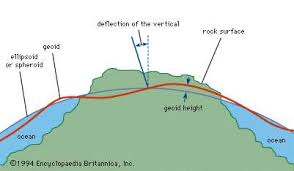The image above represents genoid height anomaly for an ocean basin.

To compute for genoid height anomaly for an ocean basin, one essential parameter is needed and this parameter is depth (d).

The formula for calculating genoid height anomaly for an ocean basin:

Δh ≃ 3.85d (0.7 – 0.046d)

Where:

Δh = Geoid Height Anomaly for an Ocean Basin
d = Depth

Let’s solve an example;
Find the genoid height anomaly for an ocean basin when the depth is 20.

This implies that;

d = depth = 20

Δh ≃ 3.85d (0.7 – 0.046d)
Δh ≃ 3.85(20) (0.7 – 0.046(20))
Δh ≃ 77 (0.7 – 0.919)
Δh ≃ 77 (-0.219)
Δh ≃ -16.93

Therefore, the genoid height anomaly for an ocean basin is -16.93 m.

Nickzom Calculator – The Calculator Encyclopedia is capable of calculating the genoid height anomaly for an ocean basin.

To get the answer and workings of the genoid height anomaly for an ocean basin using the Nickzom Calculator – The Calculator Encyclopedia. First, you need to obtain the app.

You can get this app via any of these means:

To get access to the professional version via web, you need to register and subscribe for NGN 2,000 per annum to have utter access to all functionalities.
You can also try the demo version via https://www.nickzom.org/calculator

Android (Paid) – https://play.google.com/store/apps/details?id=org.nickzom.nickzomcalculator
Android (Free) – https://play.google.com/store/apps/details?id=com.nickzom.nickzomcalculator
Apple (Paid) – https://itunes.apple.com/us/app/nickzom-calculator/id1331162702?mt=8
Once, you have obtained the calculator encyclopedia app, proceed to the Calculator Map, then click on Geology under Add-on.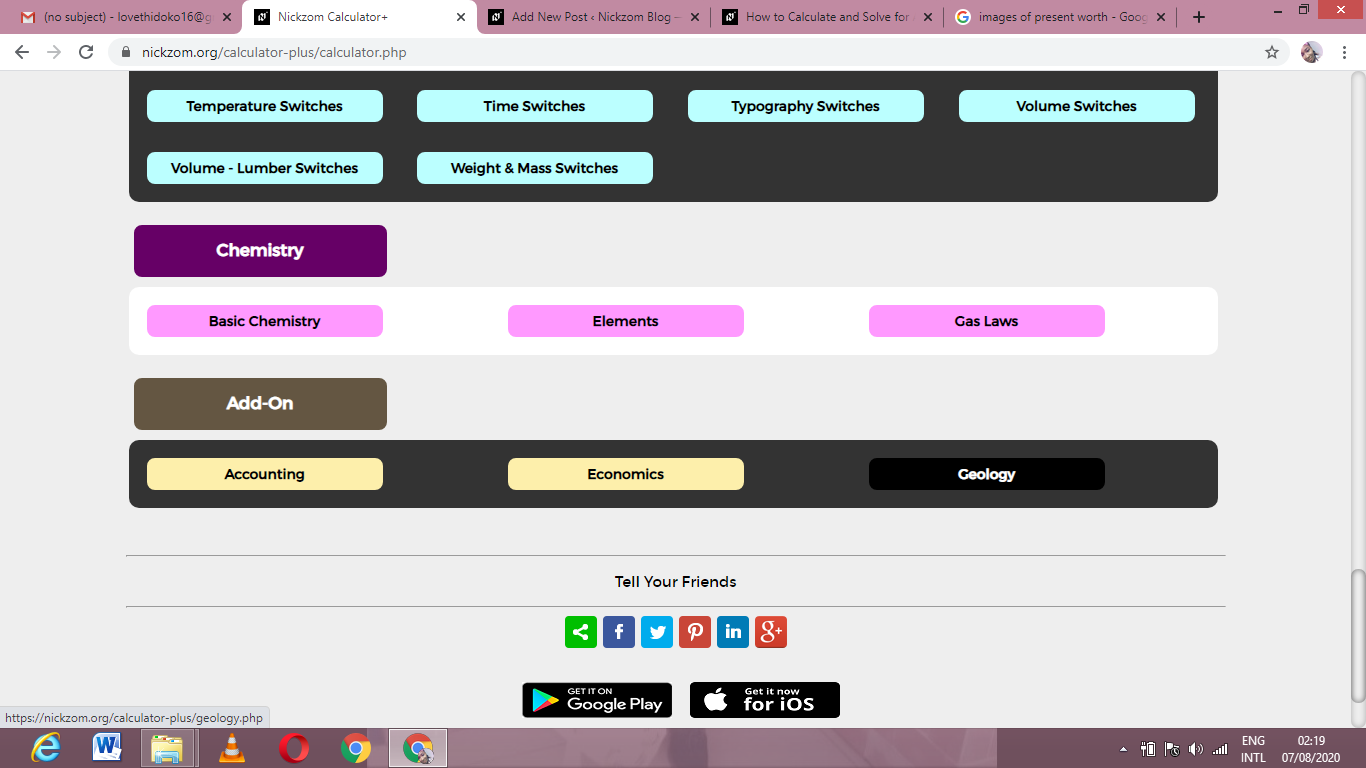Now, Click on Gravity under Geology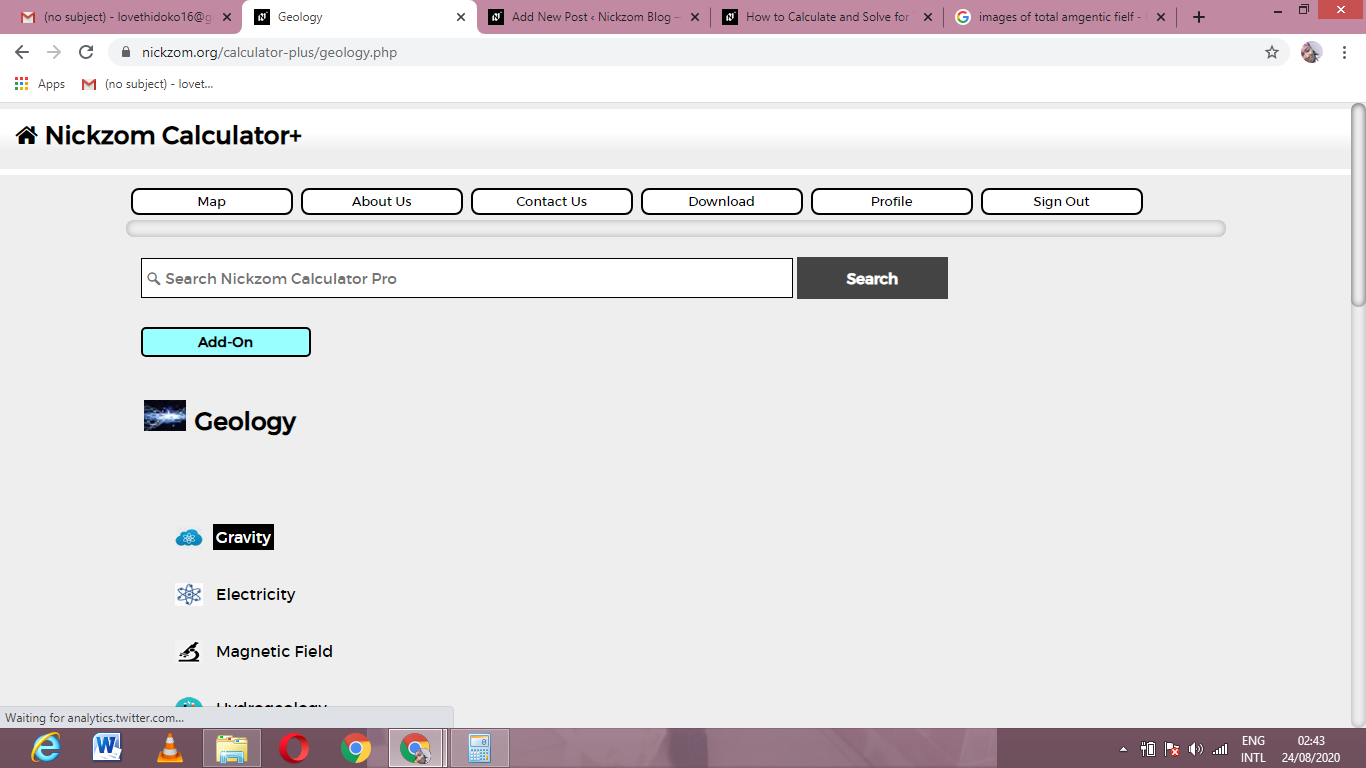Now, Click on Genoid Height Anomaly for an Ocean Basin under Gravity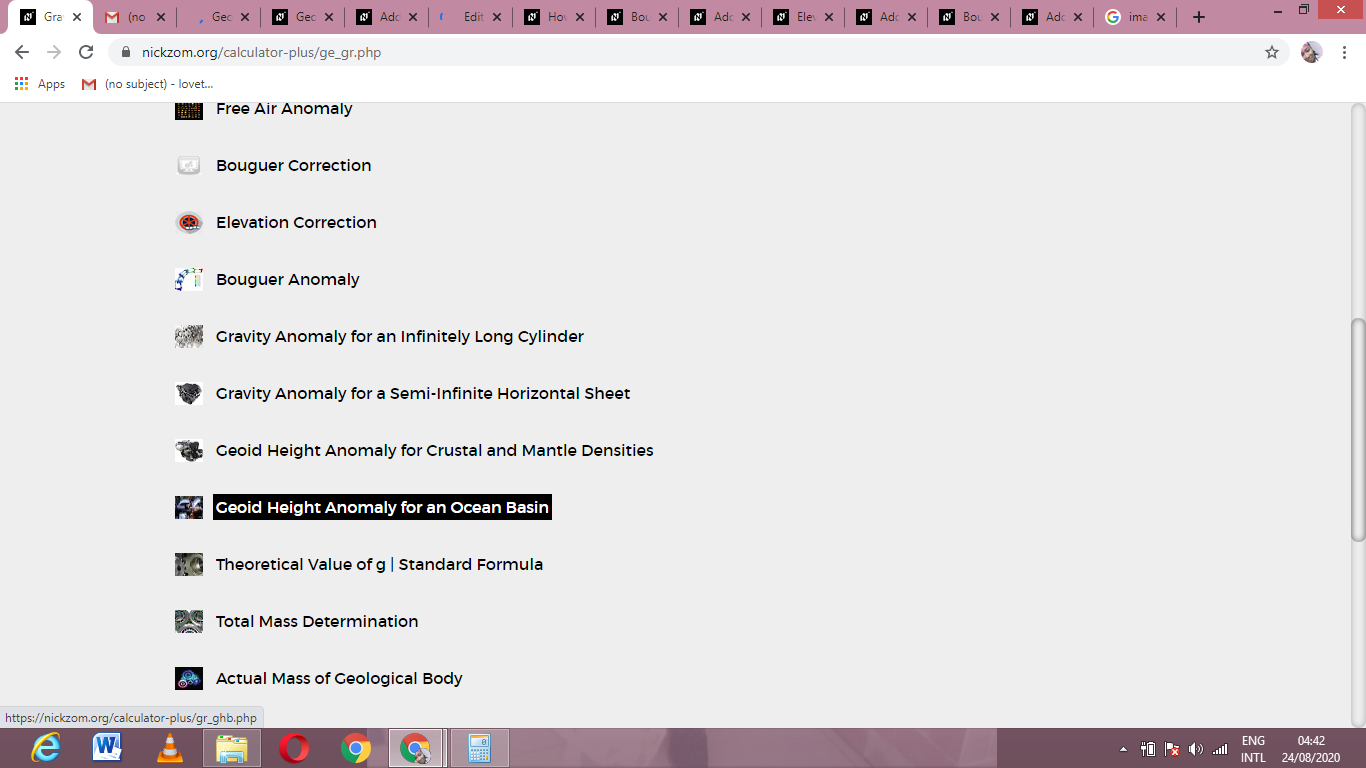The screenshot below displays the page or activity to enter your value, to get the answer for the genoid height anomaly for an ocean basin according to the respective parameter which is the depth (d).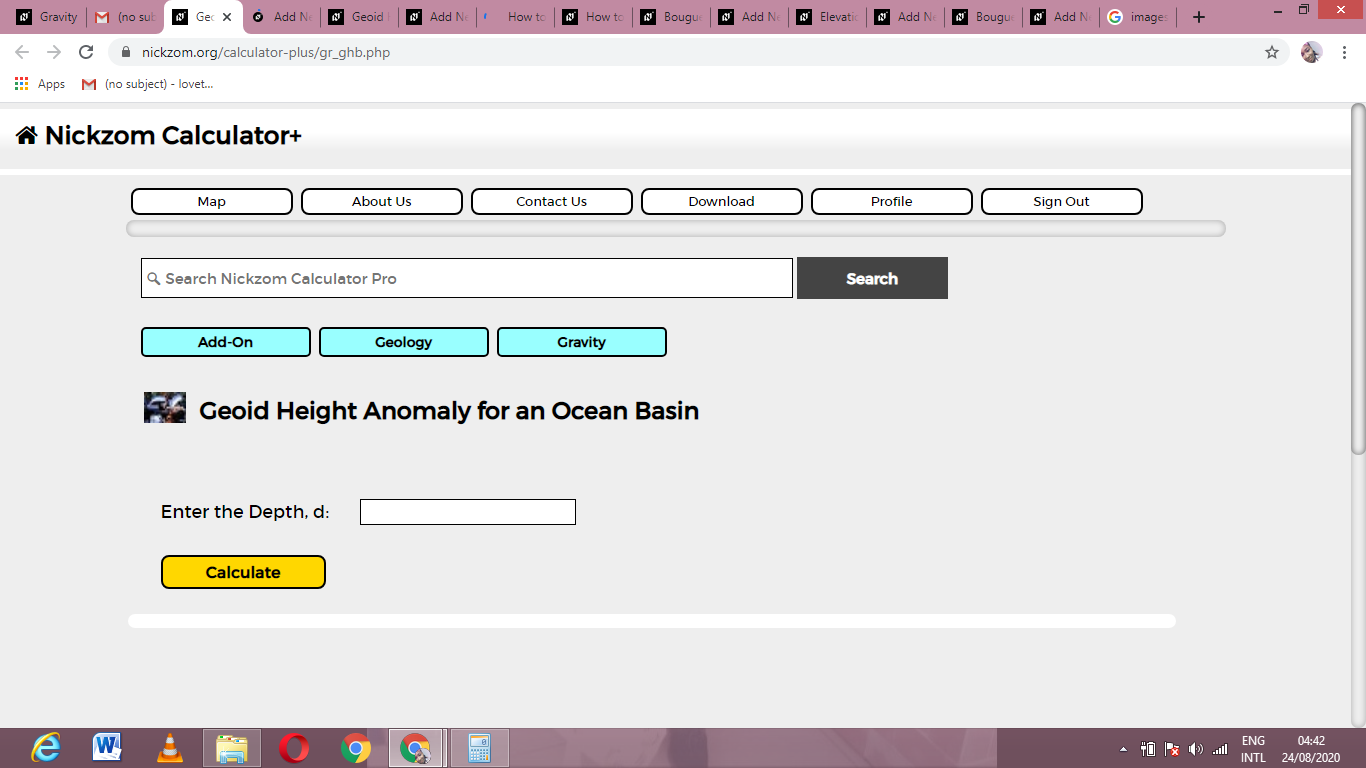Now, enter the value appropriately and accordingly for the parameter as required by the depth (d) is 20.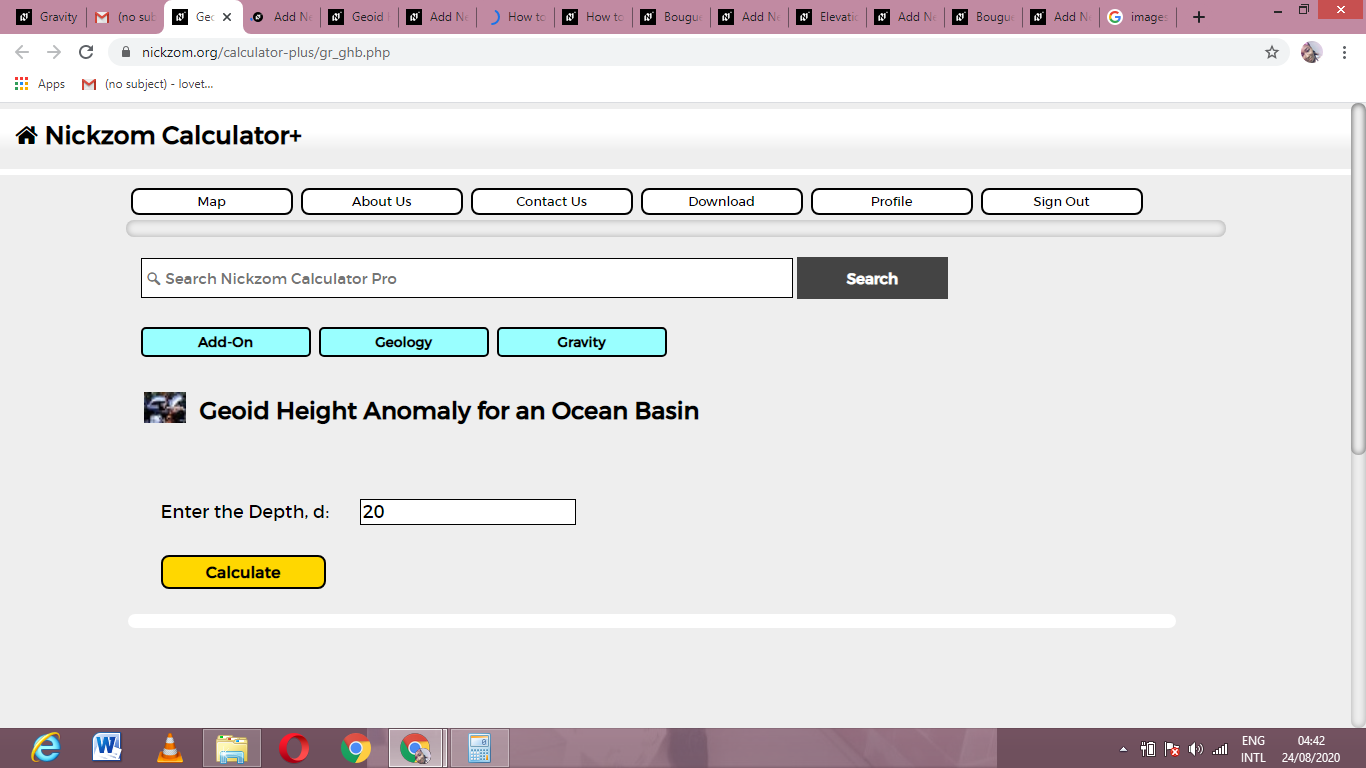Finally, Click on Calculate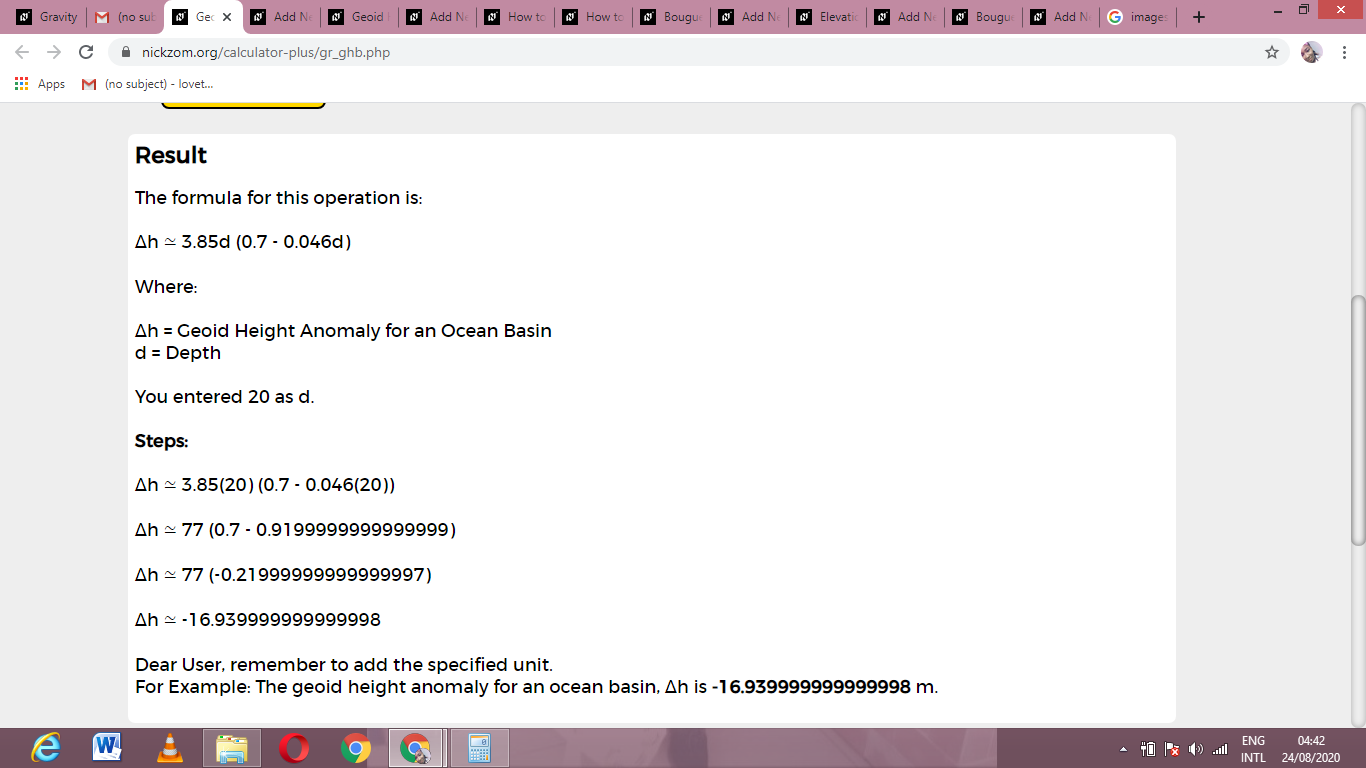As you can see from the screenshot above, Nickzom Calculator– The Calculator Encyclopedia solves for the genoid height anomaly for an ocean basin and presents the formula, workings and steps too.